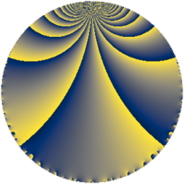# Properties

 Label 8001.2.a.jLevel $8001$ Weight $2$ Character orbit 8001.a Self dual yes Analytic conductor $63.888$ Analytic rank $1$ Dimension $2$ CM no Inner twists $1$

# Related objects

## Newspace parameters

 Level: $$N$$ $$=$$ $$8001 = 3^{2} \cdot 7 \cdot 127$$ Weight: $$k$$ $$=$$ $$2$$ Character orbit: $$[\chi]$$ $$=$$ 8001.a (trivial)

## Newform invariants

 Self dual: yes Analytic conductor: $$63.8883066572$$ Analytic rank: $$1$$ Dimension: $$2$$ Coefficient field: $$\Q(\sqrt{2})$$ Defining polynomial: $$x^{2} - 2$$ Coefficient ring: $$\Z[a_1, a_2]$$ Coefficient ring index: $$1$$ Twist minimal: no (minimal twist has level 2667) Fricke sign: $$1$$ Sato-Tate group: $\mathrm{SU}(2)$

## $q$-expansion

Coefficients of the $$q$$-expansion are expressed in terms of $$\beta = \sqrt{2}$$. We also show the integral $$q$$-expansion of the trace form.

 $$f(q)$$ $$=$$ $$q + \beta q^{2} -\beta q^{5} + q^{7} -2 \beta q^{8} +O(q^{10})$$ $$q + \beta q^{2} -\beta q^{5} + q^{7} -2 \beta q^{8} -2 q^{10} + 2 \beta q^{11} -2 q^{13} + \beta q^{14} -4 q^{16} + ( 3 - 2 \beta ) q^{17} + ( -4 + 3 \beta ) q^{19} + 4 q^{22} + 2 \beta q^{23} -3 q^{25} -2 \beta q^{26} + ( 3 + \beta ) q^{29} + 4 q^{31} + ( -4 + 3 \beta ) q^{34} -\beta q^{35} + ( 3 - 6 \beta ) q^{37} + ( 6 - 4 \beta ) q^{38} + 4 q^{40} + ( 3 - 4 \beta ) q^{41} -10 q^{43} + 4 q^{46} + ( 6 + 2 \beta ) q^{47} + q^{49} -3 \beta q^{50} + ( 9 + \beta ) q^{53} -4 q^{55} -2 \beta q^{56} + ( 2 + 3 \beta ) q^{58} -5 \beta q^{59} + ( -8 - 3 \beta ) q^{61} + 4 \beta q^{62} + 8 q^{64} + 2 \beta q^{65} -3 \beta q^{67} -2 q^{70} + ( -6 + \beta ) q^{71} + ( 2 + 9 \beta ) q^{73} + ( -12 + 3 \beta ) q^{74} + 2 \beta q^{77} -11 q^{79} + 4 \beta q^{80} + ( -8 + 3 \beta ) q^{82} + ( -6 + 4 \beta ) q^{83} + ( 4 - 3 \beta ) q^{85} -10 \beta q^{86} -8 q^{88} + ( -12 - 2 \beta ) q^{89} -2 q^{91} + ( 4 + 6 \beta ) q^{94} + ( -6 + 4 \beta ) q^{95} + ( 7 - 3 \beta ) q^{97} + \beta q^{98} +O(q^{100})$$ $$\operatorname{Tr}(f)(q)$$ $$=$$ $$2q + 2q^{7} + O(q^{10})$$ $$2q + 2q^{7} - 4q^{10} - 4q^{13} - 8q^{16} + 6q^{17} - 8q^{19} + 8q^{22} - 6q^{25} + 6q^{29} + 8q^{31} - 8q^{34} + 6q^{37} + 12q^{38} + 8q^{40} + 6q^{41} - 20q^{43} + 8q^{46} + 12q^{47} + 2q^{49} + 18q^{53} - 8q^{55} + 4q^{58} - 16q^{61} + 16q^{64} - 4q^{70} - 12q^{71} + 4q^{73} - 24q^{74} - 22q^{79} - 16q^{82} - 12q^{83} + 8q^{85} - 16q^{88} - 24q^{89} - 4q^{91} + 8q^{94} - 12q^{95} + 14q^{97} + O(q^{100})$$

## Embeddings

For each embedding $$\iota_m$$ of the coefficient field, the values $$\iota_m(a_n)$$ are shown below.

For more information on an embedded modular form you can click on its label.

Label $$\iota_m(\nu)$$ $$a_{2}$$ $$a_{3}$$ $$a_{4}$$ $$a_{5}$$ $$a_{6}$$ $$a_{7}$$ $$a_{8}$$ $$a_{9}$$ $$a_{10}$$
1.1
 −1.41421 1.41421
−1.41421 0 0 1.41421 0 1.00000 2.82843 0 −2.00000
1.2 1.41421 0 0 −1.41421 0 1.00000 −2.82843 0 −2.00000
 $$n$$: e.g. 2-40 or 990-1000 Significant digits: Format: Complex embeddings Normalized embeddings Satake parameters Satake angles

## Atkin-Lehner signs

$$p$$ Sign
$$3$$ $$-1$$
$$7$$ $$-1$$
$$127$$ $$1$$

## Inner twists

This newform does not admit any (nontrivial) inner twists.

## Twists

By twisting character orbit
Char Parity Ord Mult Type Twist Min Dim
1.a even 1 1 trivial 8001.2.a.j 2
3.b odd 2 1 2667.2.a.g 2

By twisted newform orbit
Twist Min Dim Char Parity Ord Mult Type
2667.2.a.g 2 3.b odd 2 1
8001.2.a.j 2 1.a even 1 1 trivial

## Hecke kernels

This newform subspace can be constructed as the intersection of the kernels of the following linear operators acting on $$S_{2}^{\mathrm{new}}(\Gamma_0(8001))$$:

 $$T_{2}^{2} - 2$$ $$T_{5}^{2} - 2$$

## Hecke characteristic polynomials

$p$ $F_p(T)$
$2$ $$-2 + T^{2}$$
$3$ $$T^{2}$$
$5$ $$-2 + T^{2}$$
$7$ $$( -1 + T )^{2}$$
$11$ $$-8 + T^{2}$$
$13$ $$( 2 + T )^{2}$$
$17$ $$1 - 6 T + T^{2}$$
$19$ $$-2 + 8 T + T^{2}$$
$23$ $$-8 + T^{2}$$
$29$ $$7 - 6 T + T^{2}$$
$31$ $$( -4 + T )^{2}$$
$37$ $$-63 - 6 T + T^{2}$$
$41$ $$-23 - 6 T + T^{2}$$
$43$ $$( 10 + T )^{2}$$
$47$ $$28 - 12 T + T^{2}$$
$53$ $$79 - 18 T + T^{2}$$
$59$ $$-50 + T^{2}$$
$61$ $$46 + 16 T + T^{2}$$
$67$ $$-18 + T^{2}$$
$71$ $$34 + 12 T + T^{2}$$
$73$ $$-158 - 4 T + T^{2}$$
$79$ $$( 11 + T )^{2}$$
$83$ $$4 + 12 T + T^{2}$$
$89$ $$136 + 24 T + T^{2}$$
$97$ $$31 - 14 T + T^{2}$$